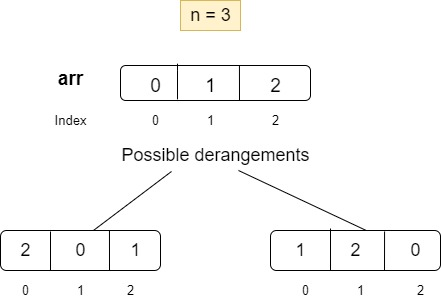New update is available. Click here to update.
Topics

# Count derangements

Moderate0/80
Average time to solve is 35m+5 more companies

## Problem statement

A Derangement is a permutation of ‘N’ elements, such that no element appears in its original position. For example, an instance of derangement of {0, 1, 2, 3} is {2, 3, 1, 0}, because 2 present at index 0 is not at its initial position which is 2 and similarly for other elements of the sequence.

Given a number ‘N’, find the total number of derangements possible of a set of 'N’ elements.

Note:
``````The answer could be very large, output answer %(10 ^ 9 + 7).
``````
Detailed explanation ( Input/output format, Notes, Images )
Constraints:
``````1 <= T <= 100
1 <= N <= 3000

Time limit: 1 sec
``````
Sample Input 1:
``````2
2
3
``````
Sample Output 1:
``````1
2
``````
Explanation of Sample Output 1:
``````In test case 1, For two elements say {0, 1}, there is only one possible derangement {1, 0}. 1 is present at index 0 and not at its actual position, that is, 1. Similarly, 0 is present at index 1 and not at its actual position, that is, 0.

In test case 2, For three elements say {0, 1, 2}, there are two possible derangements {2, 0, 1} and {1, 2, 0}. In both the derangements, no element is present at its actual position.
``````Sample Input 2:
``````2
1
4
``````
Sample Output 2:
``````0
9
``````
Explanation of Sample Output 2:
``````In test case 1, For the array = {0}, there is no possible derrangements. Hence the answer is 0 (zero).

In test case 2, For the array elements = {0, 1, 2, 3}, total 9 derrangements are possible. One of them is: { 3, 2, 1, 0}.
``````Console